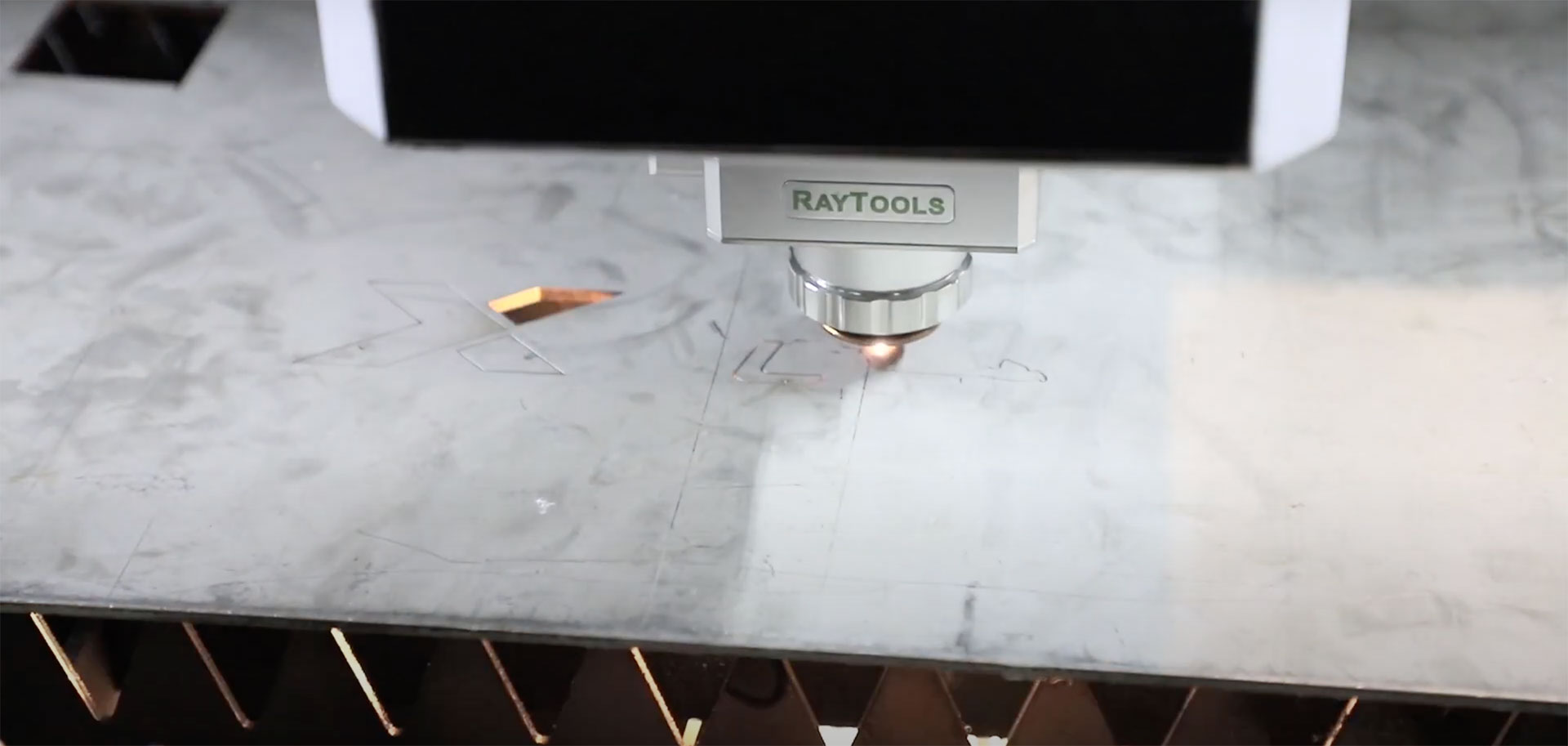×

# Laser cutting / how to calculate the production speed using the cutting perimeter speed

written April 06 2022The speed of a laser die cutter, in many cases, depends on the perimeter of the printed item (imprint).

Let’s see how we can calculate the speed in frame per minute or meters per minute.

First, here are all the input datas we need:

p: imprint perimeter

o: laser cutter output (number of imprints in a frame)

P = total perimeter (imprint perimeter x laser cutter otuput)

pp= print pitch

Vp: laser cutter speed in meters of perimeter per minute

You can calculate Vf speed of Vm speed as follow

Vf = Vp /P

Vm = Vf x pp

Let’s make an example:

We have to laser cut many circular items with a 50mm radius. The laser cutter output is 3 and its production speed in meters of perimeter is 40 mp/min. The print pitch is 100 mm.

p: imprint perimeter = 2 * pi * 50 mm = 314,15 mm

o: laser cutter output = 3

P: total perimeter = 314,15 * 3 = 942,45

pp: print pitch = 100 mm = 0,1 m

Vm: laser cutter speed in meters/min : 40 m/min

Vf and Vm Speeds:

Vf: Vp/P = 40/0,94245 = 43 f/min

Vm= Vf x pp = 43 x 0,1 = 4,3 m/min